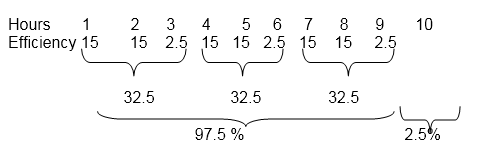# Quant Quiz – Time, Work & Wages – 25

0
(0)

Hello and welcome to exampundit. Here is a set of Quantitative Aptitude Quiz based on Time, Work & Wages problems.

1-2

Mr. Joseph employed a certain number of painters for his project. 8 days later 20% of the painters left the job and it was found that it took as much time to complete the rest work from then as the entire work needed with all the employed typists. The average speed of a painter is 20 m2 per hour .

1. How many painters left the work ?

A) 10

B) 5

C) 16

D) 2

E) Cannot be determined

Option: E

Explanation:

Let the actual number of painters be P and required number of days be D

PD=(8P)+(4P×D/5)

=>D=40

Since in the above equation , it is independent of the value of P , hence P cannot be determined, it can be of any value.

2. Minimum how many painters could be employed?

A) 10

B) 15

C) 5

D) 4

E) none

Option: C

Explanation:

Let the actual number of painters be P and required number of days be D

PD=(8P)+(4P×D/5)

=>D=40

Since 20 %= 1/5 painters left the job. So , there can be any value which is multiple of 5 1.e- whose 20% is always an integer. Hence, here 5 is the least possible answer.

3. Ekta can do 6 times the actual work in 36 days while Feena can do 1/4th of the original work in 3 days. In how many days will both working together complete the 3 times original work?

A) 6

B) 12

C) 10

D) 15

E) None

Option: B

Explanation:

Efficiency of Ekta =16066%

Efficiency of Feena= 8.33%

Total efficiency = 25%

So , they can do original work in 4 days

3 times more work requires 4×3=12 days

4. An inlet pipe can fill a tank in 5 hours and an outlet tank can empty in 36 hours, working alone. How many additional outlets of the same capacity need to be opened so that the tank never overflows?

A) 3

B) 8

C) 6

D) 7

E) 12

Option: C

Explanation:

One inlet = 7.2 times efficient of outlet

In order to prevent overflow total 8 outlets are required

Hence extra outlet = 8-1=7

5-7. Ramesh undertook a project to complete in 20 days which needs5 workers to work continuously for all the estimated days. But, before the start of the work, the client wanted to complete the project earlier than the scheduled date, so Ramesh calculated that he needs to increase 5 additional men every 2 days to complete the work in the new time ordered by the client.

5. How many men were working on the day the project was completed as per the client’s date?

A) 5

B) 20

C) 10

D) 15

E) None

Option: B

Explanation:

Total work= 5×20=100man days

Let the client needed to complete in n days

5×2+10×2+15×2+20×2=100

Hence in 8 days all the work will be completed as per client’s desire

And on 8th day 20 men working

6. If the work was further increased by 50 % but Ramesh continues to increase 5 workers on every 2 days, then how many more days are required over the initial time specified by the client?

A) 1

B) 2

C) 5

D) 4.5

E) None

Option: B

Explanation:

Total work now= 100+50=150 mandays

In 8 days 100 mandays work is completed.

Now on 9th and 10th day there will be 25 workers. So in 2 days they will complete the additional 25 mandays work. Thus the extra days needed is 2

7. Find the number of days in which client wanted to complete his work ?

A) 15

B) 10

C) 8

D) 4

E) Cannot be determined

Option: C

Explanation:

8 days in total are required by adding successive work forces

8. A barrel is connected with 8 pipes. Some of them are inlets and others are outlets. Each of the inlet can fill it in 8 hours individually, while each of the outlets can empty it in 6 hours. If all the pipes are kept open when the tank is full, it will take 6 hours to empty. How many are the inlet pipes?

A) 5

B) 4

C) 3

D) 2

E) Cannot be determined

Option: B

Explanation:

Let n be the inlet pipes

Outlet=7-n

(8-n)1/6-n×1/8=1/6=>n=4

Or else solve by options if your fundamentals are rigidly clear

9. A tank has 2 inlet pipes which can fill the tank in 12 hours and 15 hours alone and one outlet pie which can empty the full tank in 8 hours working alone. The inlet pipes are kept open for all the time but the outlet pipe was opened after 2 hours for one hour and then again closed for 2 hours then once again opened for one hour. This pattern of outlet pipe continued till the tank got completely filled. In how many hours was the tank filled, in this patter.

A) 8 hours 24 minutes

B) 10 hours 15 minutes

C) 9 hours 10 minutes

D) 9 hours 6 minutes

E) 9 hours

Option: C

Explanation:

Efficiency of inlet pipes= 8.33+6.66=15%

Efficiency of inlet pipes with the outlet pipe= 8.33+6.66-12.5=2.5%In 9 hours 97.5 % tank will be filled. On the 10th hour 2.5 % will be filled by inlet pipes with 15% efficiency

Thus they will take 2.5/15=1/6 hours=10 mins

Total = 9 hrs 10 mins

Regards

Team EP

Average rating 0 / 5. Vote count: 0

No votes so far! Be the first to rate this post.

We are sorry that this post was not useful for you!

Let us improve this post!

Tell us how we can improve this post?